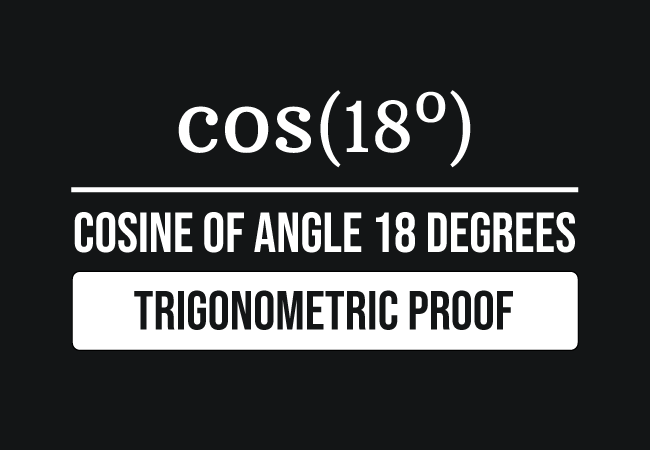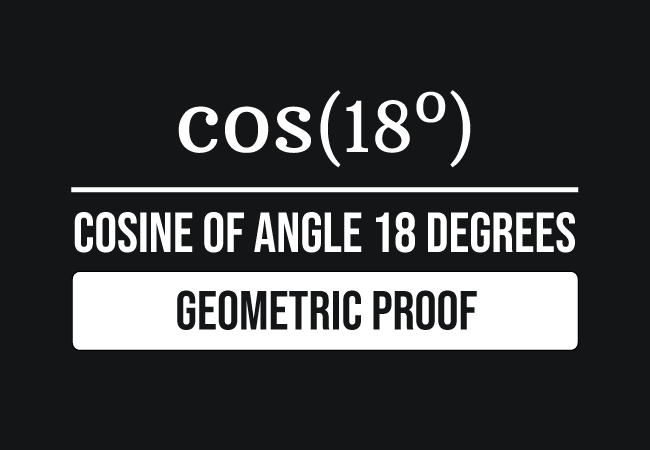# Proof for Cosine of 18 degrees

In mathematics, it is possible to derive the value of cos 18 degrees in two different methods and it is essential for you to learn both methods for knowing how to derive the cosine of angle pi by ten radian mathematically. Now, let us learn how to prove cosine of angle twenty grades in both methods.

### Trigonometric approachThe exact value for the cosine of angle eighteen degrees can be derived theoretically in the following fraction form by using a trigonometric identity.

$\cos{\big(18^\circ\big)} \,=\, \dfrac{\sqrt{10+2\sqrt{5}}}{4}$

It is actually proved in trigonometry on the basis of the sine of angle eighteen degrees. It is time to learn how to derive the cos of angle pi by ten radian exactly in trigonometric method.

### Geometrical approachThe value of the cosine of angle eighteen degrees can also be derived geometrically. It is practically possible by constructing a right triangle with an angle of eighteen degrees.

Due to the approximation in measuring the lengths of the sides, it is not possible to evaluate the cosine of angle twenty grades exactly in geometric method. However, it is possible to derive the cos of angle $18$ degrees approximately. Now, let’s learn how to derive cosine $18$ degrees value in geometry.

#### Conclusion

In trigonometric method, it is derived that the value of cosine of angle eighteen degrees is the square root of ten plus two times square root of five by four and its value in decimal form is $0.9510565162\ldots$

$\cos{\big(18^\circ\big)}$ $\,=\,$ $\dfrac{\sqrt{10+2\sqrt{5}}}{4}$ $\,=\,$ $0.9510565162\ldots$

In geometric method, it is derived that the value of cos of angle eighteen degrees in decimal form is $0.9523809523\ldots$

Actually, we have derived two values for cosine of angle eighteen degrees. It confuses us which value we have to consider as the true value for the cosine of angle twenty gradians. It can be clarified by evaluating their difference.

$( 0.9510565162\ldots )$ $\,-\,$ $( 0.9523809523\ldots )$ $\,=\,$ $-0.0013244360\ldots$

$\implies$ $( 0.9510565162\ldots )$ $\,-\,$ $( 0.9523809523\ldots )$ $\,\approx\,$ $0$

The difference between them is $-0.0013244360\ldots$, which is approximately equals to zero.

Therefore, both trigonometric and geometric methods are true for proving the value of cosine of angle eighteen degrees but the geometric method cannot give us the exact value. So, trigonometric proof is a recommendable method for deriving the cosine $18$ degrees value in mathematics.

Latest Math Topics
Jun 26, 2023
Jun 23, 2023

###### Math Questions

The math problems with solutions to learn how to solve a problem.

Learn solutions

Practice now

###### Math Videos

The math videos tutorials with visual graphics to learn every concept.

Watch now

###### Subscribe us

Get the latest math updates from the Math Doubts by subscribing us.